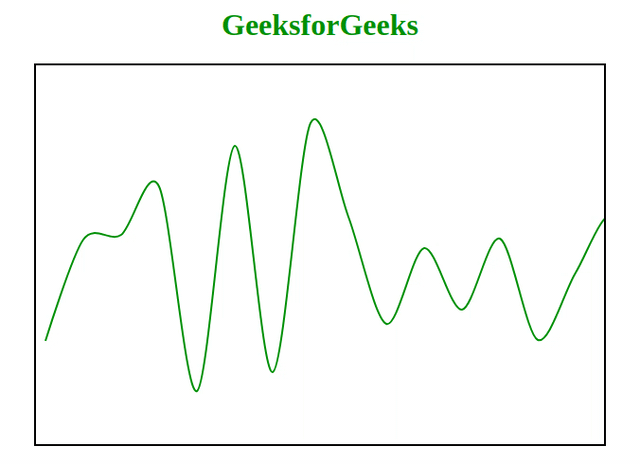# How to Draw Smooth Curve Through Multiple Points using JavaScript ?

Drawing a smooth curve with the multiple points is a challenging task. There are many algorithms that can help us to draw a curve using particular points. Bezier Curve is the one of the curve drawing technique. There is always lots of discussion around how to draw a smooth curve through the multiple numbers of points using JavaScript. So, we are given to draw a smooth curve through the multiple numbers of points.

For drawing a line we should have a slope of the line. So here we calculate the slope of a line by taking multiple inputs of x and y. For drawing a smooth curve we should have multiple numbers of inputs/points by which we can draw the smooth curve. Here we are trying to get a random number and for which we are trying to draw a smooth curve.

Here, we give you some ideas about drawing a smooth curve using multiple numbers of points. Please go through this code and we will explain it further.

Example:

 ` ` `<``html``> ` ` `  `<``head``> ` `    ``<``title``> ` `        ``Smooth Curve ` `    `` ` `     `  `    ``<``style``>  ` `        ``#GFG {  ` `            ``border: 2px solid black;  ` `        ``}  ` `        ``h1{  ` `            ``color: green;  ` `        ``}  ` `    ``  ` ` ` ` `  `<``body``> ` `    ``<``center``> ` `        ``<``h1``>GeeksforGeeks  ` `     `  `        ``<``canvas` `id``=``"GFG"` `            ``width``=``"600"` `height``=``"400"``> ` `        `` ` `    `` ` `     `  `    ``<``script``> ` `        ``var cv = document.getElementById("GFG"); ` `        ``var ctx = cv.getContext("2d"); ` `     `  `        ``function gradient(a, b) { ` `            ``return (b.y-a.y)/(b.x-a.x); ` `        ``} ` `     `  `        ``function bzCurve(points, f, t) { ` `            ``if (typeof(f) == 'undefined') f = 0.3; ` `            ``if (typeof(t) == 'undefined') t = 0.6; ` `         `  `            ``ctx.beginPath(); ` `            ``ctx.moveTo(points.x, points.y); ` `         `  `            ``var m = 0; ` `            ``var dx1 = 0; ` `            ``var dy1 = 0; ` `     `  `            ``var preP = points; ` `     `  `            ``for (var i = 1; i < ``points.length``; i++) { ` `                ``var ``curP` `= ``points``[i]; ` `                ``nexP` `= ``points``[i + 1]; ` `                ``if (nexP) { ` `                    ``m` `= ``gradient``(preP, nexP); ` `                    ``dx2 = (nexP.x - curP.x) * -f; ` `                    ``dy2` `= ``dx2` `* m * t; ` `                ``} else { ` `                    ``dx2` `= ``0``; ` `                    ``dy2` `= ``0``; ` `                ``} ` `                 `  `                ``ctx.bezierCurveTo( ` `                    ``preP.x - dx1, preP.y - dy1, ` `                    ``curP.x + dx2, curP.y + dy2, ` `                    ``curP.x, curP.y ` `                ``); ` `             `  `                ``dx1` `= ``dx2``; ` `                ``dy1` `= ``dy2``; ` `                ``preP` `= ``curP``; ` `            ``} ` `            ``ctx.stroke(); ` `        ``} ` ` `  `        ``// Generate random data  ` `        ``var lines = [];   ` `        ``var ``X` `= ``10``; ` `        ``var ``t` `= ``40``; // control the width of X. ` `        ``for (var ``i` `= ``0``; i < 100; i++ ) { ` `            ``Y` `= ``Math``.floor((Math.random() * 300) + 50); ` `            ``p = { x: X, y: Y }; ` `            ``lines.push(p); ` `            ``X` `= X + t; ` `        ``} ` ` `  `        ``// Draw smooth line  ` `        ``ctx.setLineDash(); ` `        ``ctx.lineWidth` `= ``2``; ` `        ``ctx.strokeStyle` `= ``"green"``; ` `        ``bzCurve(lines, 0.3, 1); ` `    ``  ` ` ` ` `  ` `

Output:Explanation: When we run this program, Every time it takes random inputs and this is why it generate a new smooth curve on every execution.

• Pre-requisite: HTML Canvas Basics
• Reference for canvas object is stored in variable ‘cv’ using DOM concept.
• Without having drawing context of canvas nothing can be drawn on it.
``` var cv = document.getElementById("GFG");
var ctx = cv.getContext("2d");
```
• Gradient function that return slope of the line.
```function gradient(a, b) {
return (b.y-a.y)/(b.x-a.x);
}```
• BeginPath method are used to start or reset the path.
• MoveTo method are used to move the path to the specified point in the canvas.
```  ctx.beginPath();
ctx.moveTo(points.x, points.y);
```
• Stroke is used to draw the path you have defined with all those methods.
`ctx.stroke();`

My Personal Notes arrow_drop_upCheck out this Author's contributed articles.

If you like GeeksforGeeks and would like to contribute, you can also write an article using contribute.geeksforgeeks.org or mail your article to contribute@geeksforgeeks.org. See your article appearing on the GeeksforGeeks main page and help other Geeks.

Please Improve this article if you find anything incorrect by clicking on the "Improve Article" button below.

Practice Tags :

Be the First to upvote.

Please write to us at contribute@geeksforgeeks.org to report any issue with the above content.